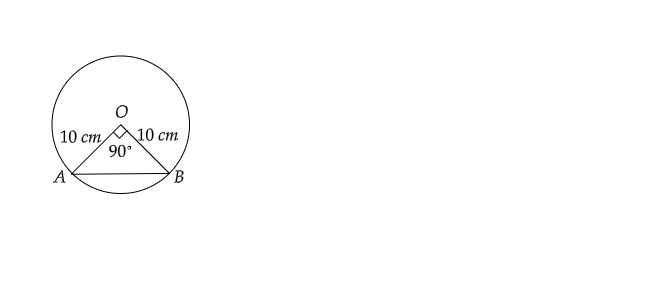# A chord of a circle of radius $10\ cm$ subtends a right angle at the center. Find the area of the corresponding minor segment.

Given: A chord of a circle of radius $10\ cm$ subtends a right angle at the center.

To do: To find the area of the corresponding minor sector.

Solution:Area of the minor sector$=$Area of the segment $AOB-$Area of $\vartriangle AOB$

$=\frac{\pi\theta}{360^{\circ}}\times OA\times OB-\frac{1}{2}\times OA\times OB$

$=\frac{3.14\times 90^{\circ}}{360^{\circ}}\times 10\times 10-0.5\times 10\times10$

$=78.5-50$

$=38.5\ cm^2$

Thus, the area of the minor sector is $38.5\ cm^2$.

Updated on: 10-Oct-2022

71 Views